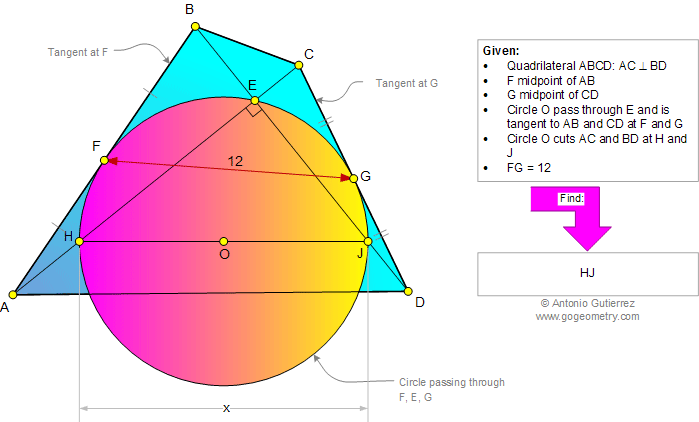# Infographic Geometry Problem 924: Quadrilateral, Diagonal, Perpendicular, Circle, Tangent, Midpoint, Metric Relations. Level: High School, College, Mathematics Education

< PREVIOUS PROBLEM  |  NEXT PROBLEM >

 In a quadrilateral ABCD with perpendicular diagonals AC and BD at E (figure below), F is the midpoint of AB and G the midpoint of CD. A circle O passing through F,E,G is tangent to AB at F and CD at G. Circle O cuts AC and BD at H and J, respectively. If FG = 12, find the measure of HJ.Home | Search | Geometry | Problems | All Problems | Open Problems | Visual Index | 10 Problems | Problems Art Gallery |  Art | 921-930 | Quadrilaterals | Triangles | Circle | Circle Tangent Line | Midpoint | Perpendicular lines | by Antonio Gutierrez